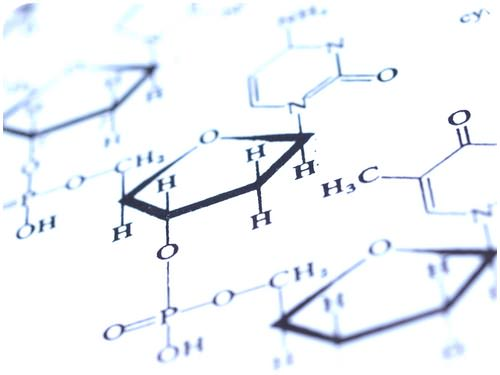# 1. 14: Biochemical ReactionsUnderstanding chemistry is essential to fully understand biology. Why?

A general understanding of chemistry is necessary to understand biology. Essentially, ourcells are just thousands of chemicals — made of elements like carbon, hydrogen, oxygen, nitrogen, phosphorus and sulfur — in just the right combinations. And these chemicals combine through chemical reactions.

## Chemical Reactions

The element chlorine (Cl) is a greenish poison. Would you eat chlorine? Of course not, but you often eat a compound containing chlorine. In fact, you probably eat this chlorine compound just about every day. Do you know what it is? It’s table salt. Table salt is sodium chloride (NaCl), which forms when chlorine and sodium (Na) combine in certain proportions. How does chlorine, a toxic green chemical, change into harmless white table salt? It happens in a chemical reaction.

A chemical reaction is a process that changes some chemical substances into others. A substance that starts a chemical reaction is called a reactant, and a substance that forms as a result of a chemical reaction is called a product. During a chemical reaction, the reactants are used up to create the products.

An example of a chemical reaction is the burning of methane. In this chemical reaction, the reactants are methane (CH4) and oxygen (O2), and the products are carbon dioxide (CO2) andwater (H2O). A chemical reaction involves the breaking and forming of chemical bonds. When methane burns, bonds break in the methane and oxygen molecules, and new bonds form in the molecules of carbon dioxide and water.

### Chemical Equations

A chemical reaction can be represented by a chemical equation. For example, the burning of methane can be represented by the chemical equation

CH4 + 2O2 → CO2 + 2H2O

The arrow in a chemical equation separates the reactants from the products and shows the direction in which the reaction proceeds. If the reaction could occur in the opposite direction as well, two arrows pointing in opposite directions would be used. The number 2 in front of O2 and H2O shows that two oxygen molecules and two water molecules are involved in the reaction. (With no number in front of a chemical symbol, just one molecule is involved.)

### Conservation of Matter

In a chemical reaction, the quantity of each element does not change; there is the same amount of each element in the products as there was in the reactants. This is because matter is always conserved. The conservation of matter is reflected in a reaction’s chemical equation. The same number of atoms of each element appears on each side of the arrow. For example, in the chemical equation above, there are four hydrogen atoms on each side of the arrow. Can you find all four of them on each side of the equation?

## Summary

• A chemical reaction is a process that changes some chemical substances into others. During a chemical reaction, the reactants are used up to create the products.
• In a chemical reaction, matter is always conserved.

## Explore More

Use this resource to answer the questions that follow.

1. What is biochemistry?
2. What is a biochemical reaction?
3. Describe the reactants and products.
4. Describe synthesis and decomposition reactions.
5. Give examples of synthesis reactions in the body.

## Review

1. Define a chemical reaction.
2. Describe the roles of reactants and products in chemical reactions.
3. How does a chemical equation show that matter is always conserved in a chemical reaction?
4. Knowing that water (H2O) forms from hydrogen (H+) and oxygen (O2), write a chemical equation for the formation of water from these two elements.